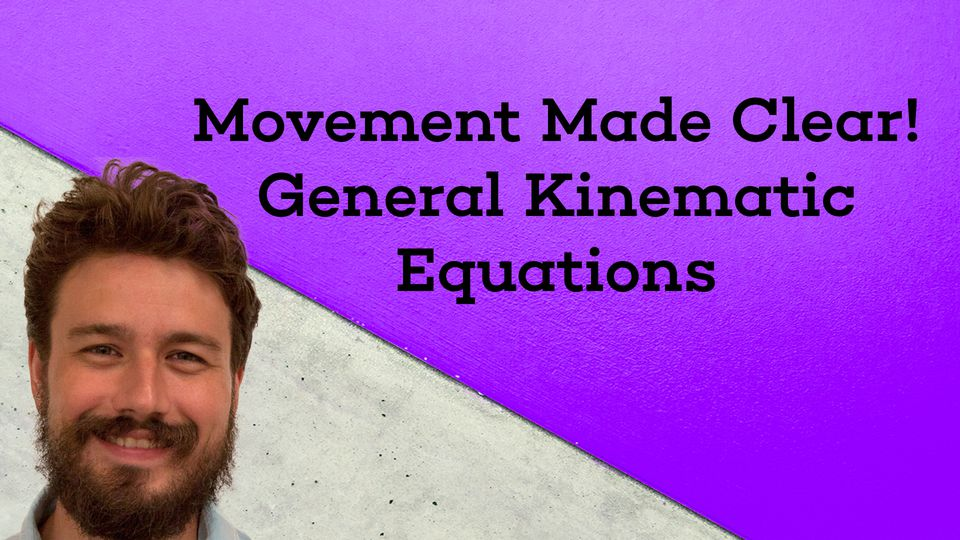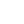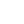Preview the Course
1h 14m

# Movement Made Clear! General Kinematic Equations

by Robert Abramovic | Science4 Lessons 1:14:37
1.1. Introduction to Kinematic Equations 16:41
2.2. The Relationship Between Position and Velocity 19:49
3.3. How Acceleration is Related to Position and Velocity 11:00
4.4. Velocity and Position Derived From Acceleration 27:07

1h 14m

Equations of motion are an important aspect of any physics course, as well as science in general. Scientists use equations describing movement to make predictions and these predictions in turn help society prepare for the future. In this course, you will learn how acceleration, velocity, and position are related via the derivative. Further, you will learn how to derive the velocity and position functions once acceleration, initial position, and initial velocity are known (using anti-derivatives and integrals). This process is applied to predicting the position and velocity of a baseball when it hits a baseball bat.

Tags:

• Equations
• Kinematics
• Motion
• Newton
• Physics
• Science
• STEM

## Instructor

Hi, I'm Robert. I hold a Ph.D. in Mathematics as well as a BA in Physics. I have been a private tutor for over 13 years. I enjoying sharing my knowledge of mathematics and physics with students, creating relatable examples, and making learning fun. My philosophy is not only teaching students but inspiring them to learn more about the material on their own.

See full profile